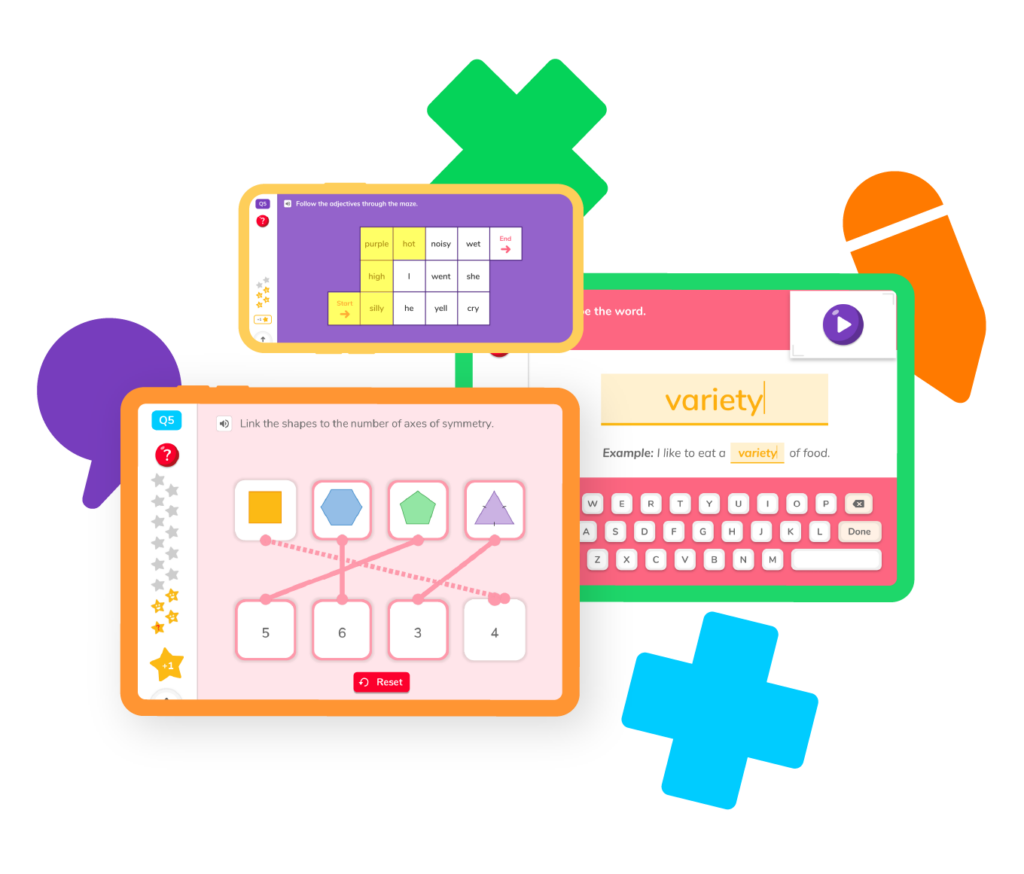4th grade math helps students build the necessary foundation for more advanced mathematical reasoning. DoodleMath has the games, guides, and review tools your student needs to not only succeed but excel in their math class. Engage in regular practice to build confidence, tackle challenges – and see math for the adventure it is!## Select a skillFractions, remainders, decimals, and factors are all important terms you’ll need to understand in order to master 4th grade math. DoodleMath has all of the guides, games, and practice exercises you need to hone your skills and tackle 4th grade math with ease.

## Multiplication

Learn all about factors and how to multiply integers together.

## Division

Discover what to do with dividends and remainders.

## Fractions

Practice with numerators, denominators, and more!

## Decimals

Make a point to dominate decimals.

## Angles

Explore every angle imaginable.

## Area and perimeter

Master calculating area and perimeter in a flash.

## Multiplication

Learn all about factors and how to multiply integers together.

## Division

Discover what to do with dividends and remainders.

## Fractions

Practice with numerators, denominators, and more!

## Decimals

Make a point to dominate decimals.

## Angles

Explore every angle imaginable.

## Area and perimeter

Master calculating area and perimeter in a flash.4th grade is an important time in your child’s life. They’re learning skills that will help them as they advance in their academic career. So what can you expect from your fourth grader’s math class, and how can you help them succeed?

Explore the skills and concepts your student will learn in 4th grade so you can guide them toward becoming the best math student they can be!

### Multiplication and division

1. Find all factors up to and including 50. Students will be able to recognize that a whole number is a multiple of its factors.
2. Solve two-step word problems with addition, subtraction, multiplication, and division. They will understand what kind of operation they must use to help them find the right answer.
3. Understand prime and composite numbers. They will be able to tell whether a number is prime (meaning it can only be divided by 1 and itself) or composite (meaning it can be factored into smaller numbers).
4. How to find the remainder when dividing numbers. Not all numbers can be divided into whole numbers. Students will learn how to identify a problem’s remainder.
5. Use all 4 operations with confidence to solve problems. By the end of 4th grade, students will know how to add, subtract, multiply, and divide whole numbers.

### Fractions

1. Understand how to equate and order fractions from largest to smallest and vice versa. Students will build on their understanding of fractions, learning how to properly order fractions and how to make them equivalent.
2. Learn how to multiply a fraction by a whole number. This will force students to combine their understanding of multiplication and fractions to solve these problems.

### Decimals

1. Use decimal notation for fractions with denominators 10 and 100. Students will learn that 6/100 equals 0.06, and how to write fractions as decimals and decimals as fractions.
2. Be able to compare decimals. Be able to analyze decimals and determine whether one is greater than, less than, or equal to another (or to a given fraction).

### Area, perimeter, and angles

1. Learn how to calculate and apply perimeter. Students will learn the perimeter formula and be able to measure the perimeter of a rectangle in both given and real-world examples.
2. Learn how to calculate and apply area. Students will learn how to calculate the area of a given shape and then use that formula to determine the area of real-world shapes.
3. Understand what an angle is. Your child will be able to see that angles are the spaces created between two rays that share a common endpoint.
4. Measure an angle’s degree using a protractor. Students will discover how to use a protractor and sketch angles of specified measurements.

Remember that all students learn at their own pace. If your student is struggling with concepts they’re learning in class, you can and should always talk to their teacher. DoodleMath allows kids to build their confidence through fun, stress-free practice. Research shows that playing math games helps students learn and retain information!While this will largely depend on the state a student lives in and the strengths of their teacher, all 4th graders are expected to go into 5th grade understanding all four major operations: addition, subtraction, multiplication, and division. They will also build upon their understanding of fractions, including how to order fractions and how to equate fractions.

Three words: practice, practice, practice! You can definitely ask their teacher for different methods and strategies to review at home. Math apps like DoodleMath allow students to play games, turning math into an adventure rather than a simple subject in school. In fact, research shows that playing math games helps with retention and builds confidence.

4th grade is when math begins to get more challenging, and if students have struggled learning addition, subtraction, multiplication, and division, then they are likely to struggle this year. There are a few signs to look for when determining whether a student needs additional help: declining grades, frustration during homework, and a sudden dislike of the subject may indicate your child needs more help.

Talk to your kid’s teacher first. They may be able to create a path toward improvement or even offer tutoring sessions before or after school. They will also know of math tutors who excel at teaching 4th graders especially.# Are you a parent, teacher or student?

Are you a parent or teacher?

## Hi there!

Book a chat with our team